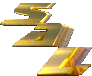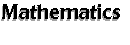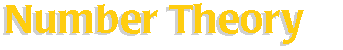[an error occurred while processing this directive] This section has several Number Theory software. Search SAL: Number Theory   (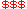Commercial,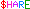Shareware,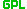GPL) CLN-- a C++ class library for numbers. ECPP -- a software package for primality proving. KANT/KASH -- computational algebraic number theory. LiDIA -- C++ library for computational number theory. Number Theoretic bc-- a fast prototyping scripting language for use in number theoretic applications. NTL-- a library for doing number theory. Pari/GP-- formal computations on recursive types at high speed. SIMATH -- computer algebra system for number theoretic purposes. Also Check Out: Algae-- a high-level interpreted language for numerical analysis. ARIBAS-- an interactive interpreter for big integer and multi-precision floating point arithmetic. MAGMA -- a system for algebra, number theory, geometry and combinatorics. Other Resources on the Internet Things of interest to number theorists http://www.maths.uq.oz.au/~krm/listi.htmlStatistics   |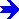Miscellaneous Software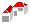SAL Home   |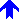Mathematics